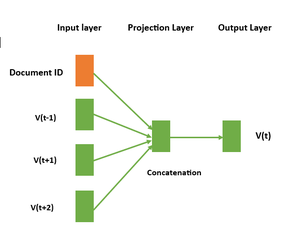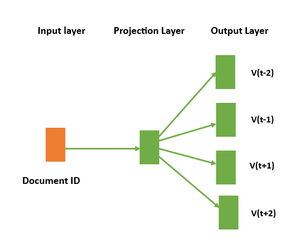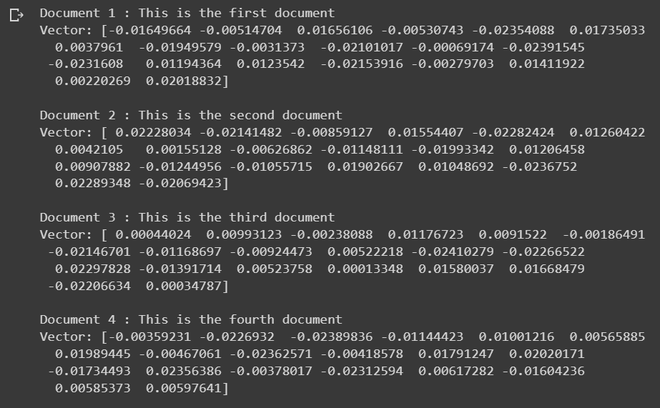# Doc2Vec in NLP

Doc2Vec is also called a Paragraph Vector a popular technique in Natural Language Processing that enables the representation of documents as vectors. This technique was introduced as an extension to Word2Vec, which is an approach to represent words as numerical vectors. While Word2Vec is used to learn word embeddings, Doc2Vec is used to learn document embeddings. In this article, we will discuss the Doc2Vec approach in detail.

## What is Doc2Vec?

Doc2Vec is a neural network-based approach that learns the distributed representation of documents. It is an unsupervised learning technique that maps each document to a fixed-length vector in a high-dimensional space. The vectors are learned in such a way that similar documents are mapped to nearby points in the vector space. This enables us to compare documents based on their vector representation and perform tasks such as document classification, clustering, and similarity analysis.

There are two main variants of the Doc2Vec approach:

• Distributed Memory (DM)
• Distributed Bag of Words (DBOW)

## Distributed Memory (DM)

Distributed Memory is a variant of the Doc2Vec model, which is an extension of the popular Word2Vec model. The basic idea behind Distributed Memory is to learn a fixed-length vector representation for each piece of text data (such as a sentence, paragraph, or document) by taking into account the context in which it appears.DM Architecture

In the DM architecture, the neural network takes two types of inputs:  the context words and a unique document ID. The context words are used to predict a target word, and the document ID is used to capture the overall meaning of the document. The network has two main components:  the projection layer and the output layer.

The projection layer is responsible for creating the word vectors and document vectors. For each word in the input sequence, a unique word vector is created, and for each document, a unique document vector is created. These vectors are learned through the training process by optimizing a loss function that minimizes the difference between the predicted word and the actual target word. The output neural network takes the distributed representation of the context and predicts the target word.

## Distributed Bag of Words (DBOW)

DBOW is a simpler version of the Doc2Vec algorithm that focuses on understanding how words are distributed in a text, rather than their meaning. This architecture is preferred when the goal is to analyze the structure of the text, rather than its content.DBOW Architecture

In the DBOW architecture, a unique vector representation is assigned to each document in the corpus, but there are no separate word vectors.  Instead, the algorithm takes in a document and learns to predict the probability of each word in the document given only the document vector.

The model does not take into account the order of the words in the document, treating the document as a collection or “bag ” of words. This makes the DBOW architecture faster to train than DM, but potentially less powerful in capturing the meaning of the documents.

## Difference between DM and DBOW

DM architecture considers both the word order and document context, making it more powerful for capturing the semantic meaning of documents, while DBOW architecture is simpler and faster to train, and is useful for capturing distributional properties of words in a corpus.

The choice between the two architectures depends on the specific goals of the task at hand,  and often both architectures are used in combination to capture both the semantic meaning and distributional properties of texts. Let’s write a Python code to implement Doc2Vec using Python’s Gensim library.

## Python3

 `from` `gensim.models.doc2vec ``import` `Doc2Vec,\` `    ``TaggedDocument` `from` `nltk.tokenize ``import` `word_tokenize`   `# define a list of documents.` `data ``=` `[``"This is the first document"``,` `        ``"This is the second document"``,` `        ``"This is the third document"``,` `        ``"This is the fourth document"``]`   `# preproces the documents, and create TaggedDocuments` `tagged_data ``=` `[TaggedDocument(words``=``word_tokenize(doc.lower()),` `                              ``tags``=``[``str``(i)]) ``for` `i,` `               ``doc ``in` `enumerate``(data)]`   `# train the Doc2vec model` `model ``=` `Doc2Vec(vector_size``=``20``,` `                ``min_count``=``2``, epochs``=``50``)` `model.build_vocab(tagged_data)` `model.train(tagged_data,` `            ``total_examples``=``model.corpus_count,` `            ``epochs``=``model.epochs)`   `# get the document vectors` `document_vectors ``=` `[model.infer_vector(` `    ``word_tokenize(doc.lower())) ``for` `doc ``in` `data]`   `#  print the document vectors` `for` `i, doc ``in` `enumerate``(data):` `    ``print``(``"Document"``, i``+``1``, ``":"``, doc)` `    ``print``(``"Vector:"``, document_vectors[i])` `    ``print``()`

Output:Document Vectors generated by Doc2Vec Model